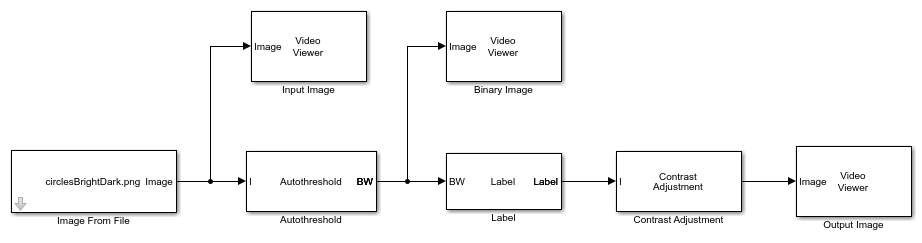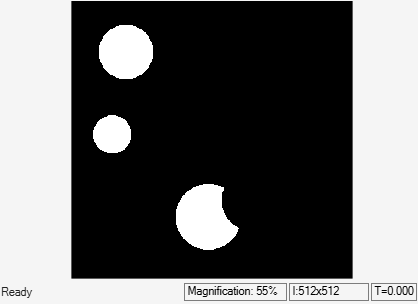# Label Objects in Binary Image

This example shows you how to label objects in a binary image.

### Example Model

```modelname = 'ex_blkLabel.slx'; open_system(modelname) ```The model reads an input intensity image using the Image From File block. The Autothreshold block converts the intensity image into a binary image using a Threshold scaling factor parameter value of `3`. To label the objects in the binary image, the model uses the Label block with these nondefault parameters:

• Output`Label matrix`

• Output data type`uint8`

To visualize the labeled objects, the Contrast Adjustment block adjusts the range of intensity values of the output label matrix of the Label block.

### Simulate and Display Results

Run the model. The model displays the input image, the binary image, and the output image using Video Viewer blocks. Observe how the output image shows three labeled objects using different shades of gray.

```sim(modelname); ```